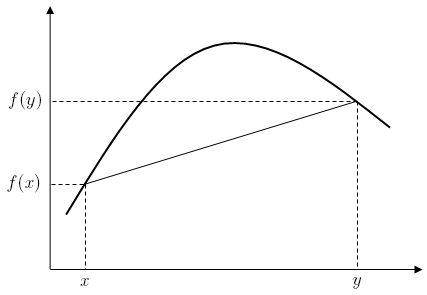# Concave function

In mathematics, a concave function is the negative of a convex function. A concave function is also synonymously called concave downwards, concave down, convex upwards, convex cap or upper convex.

## Definition

A real-valued function f on an interval (or, more generally, a convex set in vector space) is said to be concave if, for any x and y in the interval and for any α in [0,1],A function is called strictly concave iffor any α in (0,1) and xy.

For a function f:RR, this definition merely states that for every z between x and y, the point (z, f(z) ) on the graph of f is above the straight line joining the points (x, f(x) ) and (y, f(y) ).A function f is quasiconcave if the upper contour sets of the functionare convex sets.:496

## Properties

1. A function f is concave over a convex set if and only if the function −f is a convex function over the set.

2. A differentiable function f is concave on an interval if and only if its derivative function f is monotonically decreasing on that interval, that is,: a concave function has a decreasing slope.

3. Points where concavity changes (between concave and convex) are inflection points.

4. The sum of two concave functions is itself concave and so is the pointwise minimum of two concave functions, i.e. the set of concave functions on a given domain form a semifield.

5. Near a local maximum in the interior of the domain of a function, the function must be concave; as a partial converse, if the derivative of a strictly concave function is zero at some point, then that point is a local maximum.

6. If f is twice-differentiable, then f is concave if and only if f is non-positive (or, if the acceleration is non-positive). If its second derivative is negative then it is strictly concave, but the opposite is not true, as shown by f(x) = x4.

7. Any local maximum of a concave function is also a global maximum. A strictly concave function will have at most one global maximum.

8. If f is concave and differentiable, then it is bounded above by its first-order Taylor approximation::4899. A continuous function on C is concave if and only if for any x and y in C10. If a function f is concave, and f(0) ≥ 0, then f is subadditive. Proof:

• since f is concave, let y = 0,•## Examples

• The functionsandare concave on their domains, as their second derivativesandare always negative.
• The logarithm functionis concave on its domain, as its derivativeis a strictly decreasing function.
• Any affine functionis both concave and convex, but not strictly-concave nor strictly-convex.
• The sine function is concave on the interval.
• The function, whereis the determinant of a nonnegative-definite matrix B, is concave.
• Practical example: rays bending in computation of radiowave attenuation in the atmosphere.